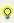Formula editor

With the formula editor – accessible via the button – it is possible to define boolean formulas for stati of the combined element. With the button in the Formula variables area it is also possible to add other variables.

The meaning of the single bits is the following:

Bit

Description

value bits

32 value bits (from 0 to 31) describing the variable value bitwise are available. For BOOL variables only bit 0 is of importance, for SINT and USINT only the bits from 0 to 7, etc. Please be aware, that the evaluation always refers to the raw value (signal resolution) of the variable and not to the scaled measuring range.

status bits

Here you find the most commonly used status bits. You will find a description of the status bits in the chapter Status administration.

not acknowledged

Not acknowledged is treated like a usual status bit. But here it is listed separately, because it does not belong to the classical variable stati.

value and status

In the formulas all values (value bits and status bits) are treated as binary values and can be logically linked with AND, OR, etc.
Exceptions are the (complete) value and the (complete) status.
In order to get a Boolean result this total value has to be ORed with a constant bitwise. For this we use the operator &.
For the result 0 (false) of this logical ORing we get the binary value 0 (false) otherwise 1 (true).
Example: You want to find out, if one of the user status bits 1 – 8 of the variable X01 is set:
Usual formula:
X01.ST_M1 OR X01.ST_M2 OR X01.ST_M3 OR X01.ST_M4 OR X01.ST_M5 OR X01.ST_M6 OR X01.ST_M7 OR X01.ST_M8
This query can be made much easier by bitwise OR-links of the (Complete)Status. The formula then is:
X01.Status & 0xFF
The constant has to be entered hexadecimally as described above. 0xFF is decimal 256, that are the first eight status bits (binary 11111111). If one of these bits is 1, the result of this bitwise ORing is 1 (TRUE) otherwise 0 (FALSE).
If e.g. all user status bits except the user status bit M7 should be queried, the binary statement would be: 10111111 (bit 7 is not interesting, so it is 0), this is hexadecimal 0xBF. The formula then is:
X01.Status & 0xBF.

Beside a bitwise OR with a constant the values also can be compared directly with a decimal value. If the comparison is wrong, the binary value is 0 (false) otherwise 1 (true).
Example: You want to compare, if the value is equal to the constant 202:
The formula then is:
X01.Wert = 202
If the value is equal to the constant 202, the result of the comparison is 1 (true) otherwise 0 (false).

The operator priority of the formula calculation is:

Priority

Bediener

1

& (the functionality of this operator is explained above under value and status)

2

NOT

3

AND

4

XOR/ORInfo Up to 99 variables can be used in one formula. X01 to X99.Info The status bits NORM and ABNORM are here only available in the formula editor. They cannot be engineered using the status.